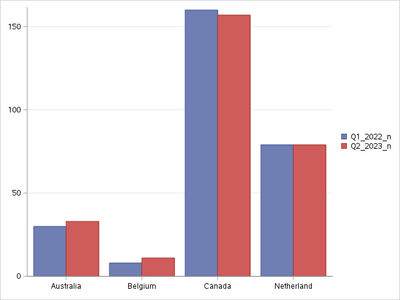## Combine n and percent for a bar graph

I am trying to display the labels on my bar graph with n and percent combined. Using SAS support community

I was able to locate this solution:

The solution works great the problem is when the percent are transposed the values are not coming out as they should. I also have several observation

and some percent's get numeric values with many decimal places (1.6198704104) when I would like 1.6.

My data is as follows:

data bar;
infile datalines dlm=',';
input Country \$ Q1_2022_n Q1_2022_pct Q2_2023_n Q2_2023_pct num_change pct_change;

datalines;
Australia,30, 1.6, 33, 1.8, 3, 0.2
Belgium, 8, 0.4, 11, 0.6, 3, 0.2
Canada, 160, 8.7, 157, 8.7, -3, 0.0
;
run;

So for the first line when the variables are combined I would like 30(1.6), 33(1.8) and 3(0.2)  instead

I get 30(10.8), 33(11.8) and 1.8(11.6). My code so far is below.

`data bar; infile datalines dlm=',';length country \$10.;input Country \$	Q1_2022_n	Q1_2022_pct	Q2_2023_n	Q2_2023_pct	num_change	pct_change;datalines; Australia,30, 1.6, 33, 1.8, 3, 0.2Belgium, 8, 0.4, 11, 0.6, 3, 0.2Canada, 160, 8.7, 157, 8.7, -3, 0.0Netherlands, 79, 4.3, 79, 4.4, 0, 0.1;run;proc sort data = bar;	by country;run; proc transpose data=bar out=bar_long(rename=(_NAME_=Group COL1=value));   by country;run;proc sql;   create table bar_cat as   select *,          cats(value, "(", put(value/(sum(value)), percent10.1), ")") as datalabel   from bar_long   group by Group;quit;`

Any assistance would be great. Thanks in advance.

4 REPLIES 4

## Re: Combine n and percent for a bar graph

First tell us, as in show the specific values and for which variable you use that gets you a desired value of 30(1.6). You should also at least mention should be Australia and Q1_2022_n. "First doesn't cut it because when I run the code you provide Australia is the third observation in the final set.

There are about eleventy-thousand ways to make bar graphs. You didn't provide any details. Vertical or horizontal? Which variable is for which axis? Clustered or stacked? Which variable would be used to identify which segment in any graph? I am guessing the Value variable is supposed to be involved with the length of the bars but are the N, percents and change all supposed to be one bar?

And a suggestion for building that n and percent value. The following code places a space between the n and the left ( as that is tad easier to read. This also uses the PercentN format. This places a negative sign in the value instead of a set of () around negative percent values. The doubled parentheses for (( 100.0%)) is a bit odd.

```proc sql;
create table bar_cat as
select *,
catx(' ',value,cats( "(", put(value/(sum(value)), percentn10.1 -L)), ")") as datalabel
from bar_long
group by Group;
quit;```

## Re: Combine n and percent for a bar graph

The values are grouped in this manner:

q1_2022_n (q1_2022_pct)

q2_2023_n (q2_2023_pct)

I would like to create a vertical cluster bar graph with countries on the xaxis; y axis would represent n for q1_2022_n and q2_2023_n by country. Above the bars would be the label for n(%)..

## Re: Combine n and percent for a bar graph

`As an example I would like a graph similar to this: `but have the data labels include n(%).

`proc sgplot data=bar_long noautolegend noborder;   vbar country / response=value group=group groupdisplay=cluster /*datalabel=datalabel*/;   keylegend / position=e title="" noborder;   yaxis grid display=(nolabel);   xaxis display=(nolabel);run;`

## Re: Combine n and percent for a bar graph

@luvscandy27 wrote:

The values are grouped in this manner:

q1_2022_n (q1_2022_pct)

q2_2023_n (q2_2023_pct)

I would like to create a vertical cluster bar graph with countries on the xaxis; y axis would represent n for q1_2022_n and q2_2023_n by country. Above the bars would be the label for n(%)..

One way to do the plot:

```proc sgplot data=bar_cat;
where group in ('Q1_2022_n' 'Q2_2023_n') ;
vbar country / response=value group=group groupdisplay=cluster
datalabel=datalabel
;
label group='Quarter'
value='Total N'
;
run;```

Depending on the amount of text in the datalabel variable, current size setting of your graphic area, active ODS style and possibly other options the text at the bar top may try to display as wider than the bar and possibly wrap text.

You could address those issues with either or both of the DatalabelAttrs to specify a smaller font size, or/and DATALABELFITPOLICY=Rotate to have the text rotated above the bar or use the Splitchar=' ' and Datalabelfitpolicy=Splitalways which would separate the N and percent vertically and appear something like this:(just made up number).

```  70
(24.8%)```

Discussion stats
• 4 replies
• 189 views
• 0 likes
• 2 in conversation